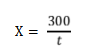# Applications

## Method for the Determination of Diastase Number in Honey by UV-Vis Spectrophotometer

Principle
After putting starch solution into honey sample solution, some starch is hydrolysis by diastase in honey. The starch residue appears as bluish violet color after mixing with iodine. As the reaction process, the color disappears finally. By using UV-Vis Spectrophotometer at 660nm wavelength, the time required to reach the specific absorbance can be measured. Therefore, the volume (ml) that 1% starch sample is hydrolyzed by 1g of honey in 1h can be calculated.

Reagents and Material
Excepts those with specific notification, AR degree reagents are used; water is ultrapure water.

Reagent:
1. Iodine.
2. Potassium Iodide.
3. Sodium Acetate (CH3COONa ·3H2O).
4. Iced Acetate Acid
5. Sodium Chloride.
6. Soluble Starch.
7. Iodine stock solution: weigh 8.8g iodine and 22g potassium iodide, mixing with 30mL~40mL water, dilute to 1000mL.
8. Iodine solution: weigh 20g potassium iodine, add 5mL iodine stock solution, dilute to 500mL by water (this solution can be only used for 2 days).
9. Acetate buffer solution: pH 5.3 (1.59 mol/L). Weigh 87g Sodium Acetate and dilute in 400mL water. Add 10.5mL iced acetate acid and dilute to 500mL. If necessary, use sodium acetate or acetate acid to regulate the pH value to 5.3.
10. Sodium chloride solution (0.5 mol/L): weigh 14.5g NaCl, dissolve in 500mL of water.
11. Starch solution: dissolve 2g soluble starch in 90mL water. Boiling the starch solution for 3min, then wait for it cooling down. Transfer the solution into a 100mL volumetric flask and dilute to 100mL with water.

Equipment:
1. UV-Vis Spectrophotometer.
2. Constant Temperature Cell Holder

Sample Preparation and Preserve
Sample Preparation:
Stir the sample well, then transfer 0.5kg into a sample bottle as testing sample. Seal and label the sample.
Note: Whether crystal appears or not, all the sample cannot be heated.

Sample Preserve:
Sample can be preserved under room temperature.

Experiment Procedure
Standard Test of Starch Solution
Measure 5.0 mL of starch solution (Reagent 11) and 10.0 mL of water, place in 40℃water bath separately for 15min. Mix the heated starch solution and heated water, then transfer 1mL of mixed solution into 10mL iodine solution (Reagent 8), dilute with certain amount of water and mix well again. Use ultrapure water as blank reference. Measuring the absorbance at 660nm wavelength by UV-Vis spectrophotometer. Record the volume of water needed to produce 0.760±0.2 absorbance. Use this volume as sample dilution coefficient. (If starch source is changed, this standard needs to be remeasured).

Sample Testing:
1. Spectrophotometer Conditions:
a) Wavelength: 660nm
b) Reference: Water
2. Sample Pretreatment: measure 5g (±0.01g) prepared sample and transfer into a 20mL beaker. Add 15mL water and 2.5mL acetate buffer solution (Reagent 9) in the beaker, then mix with 1.5mL sodium chloride solution (Reagent 10) in a 25mL volumetric flask and dilute to 25mL with water.
3. Measure 5mL starch solution, 10mL sample solution (Sample Testing 2), and 10mL iodine solution, place in 40℃water bath separately for 15min. Transfer the heated starch solution into the heated sample solution and mix well.
4. After 5min, transfer 1.0mL mixed solution into 10mL heated iodine solution, and dilute with the recorded amount of water (recorded in Standard Test of Starch Solution step) and mix well again. Measure the absorbance of sample at 660 nm wavelength with the same water blank by UV-Vis Spectrophotometer.
5. If the absorbance is greater than 0.235, the 4th step need to be repeated until the absorbance is less than 0.235.
6. During measurement, the mixed solution, iodine solution and water need to be maintained at 40℃water bath. Sample during measurement needs to be put in Constant Temperature Cell Holder.

Calculation
On a logarithmic coordinate paper, name y-axis as absorbance (%), and x-axis as time (min). Put spots on the paper with the measured absorbance and its correspondence time. Connect all the dots as a line, find the time in which the absorbance of sample and 0.235 intersect. Finally, the diastase number can be calculated by the formula:Where X is the diastase number in solution (mL/(g ·h)), t is the corresponding time (min). The calculated number should round to one decimal place.

Results
By using this method, the repeatability and reproducibility of the experiment are ≥95%.

## media contact

Meet With PRofessionals